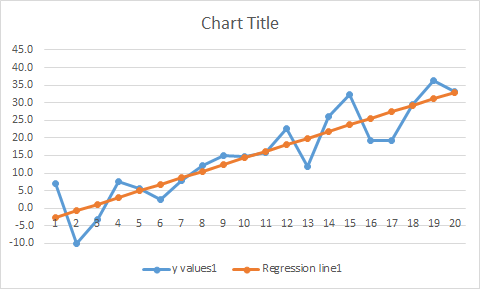11
Aug 16

## The pearls of AP Statistics 18

Better see once than hear a thousand times: the error in regression model

They say: The regression line is introduced in Chapter 2 of Agresti and Franklin. The true regression model$y=a+bx+e$ is never mentioned (here$e$ is the error term). In Chapter 12 (p.583) the existence of the error term is acknowledged in section "The Regression Model Also Allows Variability About the Line" and Figure 12.4.

I say: the formal treatment of the true model, error term and their implications for inference is beyond the scope of this book. The informal understanding can be enhanced by the following illustrations. In both cases the true intercepts, slopes, sigmas and error distributions are the same. The differences between the observations and regression lines are caused by randomness. Download the Excel file with simulations. Press F9 to see different realizations.

Simulations steps:

1. The user can change the intercept, slope and sigma to his/her liking.
2. The x values are just natural numbers.
3. The errors are obtained from rand() by centering and scaling
4. The y values are generated using the regression formulas
5. The estimated slope and intercept are Excel functions
6. They are used to calculate the fitted values
7. For the second sample steps 3-6 are repeatedFigure 1. Regression line and observations for sample 1Figure 2. Regression line and observations for sample 2Figure 3. Comparison of two regression lines